Get inspired by the success stories of our students in IIT JAM MS, ISI  MStat, CMI MSc DS.  Learn More

# ISI MStat PSB 2011 Problem 1 | Linear Algebra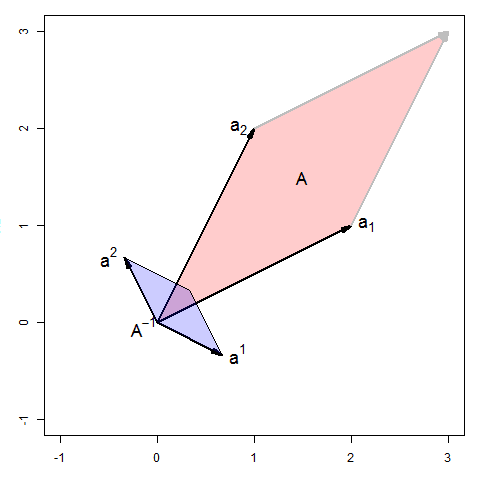This is a quite pleasant sample problem from ISI MStat PSB 2011 Problem 1. It is mainly about, patterns in matrices and determinants and using a special kind of determinant decomposition, which is widely used in Statistics . Give it a try !

## Problem- ISI MStat PSB 2011 Problem 1

Let A be a nxn matrix, given as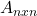= \begin{pmatrix} a & b & \cdots & b \\b& a & \cdots & b \\ \vdots & \vdots & \ddots & \vdots \\ b & b & \cdots & a \end{pmatrix} ;

where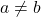and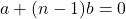.

Suppose B=A+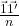where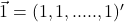is nx1 vector.

Show that,

(a) B is non-singular .

(b)### Prerequisites

Basic Matrix multiplication

Determinants.

Matrix decomposition .

## Solution :

While attacking a problem related to matrices, the primary approach which I find very helpful is, realizing if there is any subtle pattern hidden. Similarly, here also a pattern is very much prominent exhibited in the matrix A, hence we will break A as,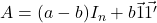.

which reduces,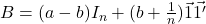.

now, we can find Determinant of B, by using a known decomposition , which follows from determinants of partitioned matrices.

we have the decomposition as for some non-singular M and column vectors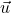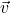,

we have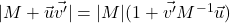, when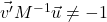.

for, this particular problem ,==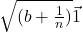,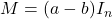, which is non-singular, and clearly,

If you are not familiar with these, then become friendly with this decomposition, as it is has very important and frequent applications in Statistics. Best to derive it !

So,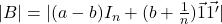=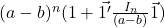=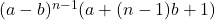=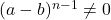asand, So, hence B is non-singular. Also, B is invertible, we will need this to do the next part

for the second part (b), observe that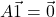why ??? , so,................(*)

So,....using(*)

now left multiplying A, to the above matrix equation, we have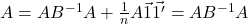. hence , we are done !!

## Food For Thought

Suppose, it is given that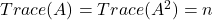, Can You show that all the eigenvalues of A are equal to 1 ? Is it true for any symmetric matrix,following the given condition? Give it a thought !

[ In case, you don't know what eigenvalues are, its a scalar,which one may find for a square matrix C, such that, for a non-null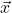,, for a matrix order n, one will find n such scalars or eigenvalues, say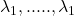,

then, and , for symmetric matrices, all eigenvalues are real, so you don't need to worry much but you can obviously verify it !! ]

## Subscribe to Cheenta at Youtube

This is a quite pleasant sample problem from ISI MStat PSB 2011 Problem 1. It is mainly about, patterns in matrices and determinants and using a special kind of determinant decomposition, which is widely used in Statistics . Give it a try !

## Problem- ISI MStat PSB 2011 Problem 1

Let A be a nxn matrix, given as= \begin{pmatrix} a & b & \cdots & b \\b& a & \cdots & b \\ \vdots & \vdots & \ddots & \vdots \\ b & b & \cdots & a \end{pmatrix} ;

whereand.

Suppose B=A+whereis nx1 vector.

Show that,

(a) B is non-singular .

(b)### Prerequisites

Basic Matrix multiplication

Determinants.

Matrix decomposition .

## Solution :

While attacking a problem related to matrices, the primary approach which I find very helpful is, realizing if there is any subtle pattern hidden. Similarly, here also a pattern is very much prominent exhibited in the matrix A, hence we will break A as,.

which reduces,.

now, we can find Determinant of B, by using a known decomposition , which follows from determinants of partitioned matrices.

we have the decomposition as for some non-singular M and column vectors,

we have, when.

for, this particular problem ,==,, which is non-singular, and clearly,

If you are not familiar with these, then become friendly with this decomposition, as it is has very important and frequent applications in Statistics. Best to derive it !

So,===asand, So, hence B is non-singular. Also, B is invertible, we will need this to do the next part

for the second part (b), observe thatwhy ??? , so,................(*)

So,....using(*)

now left multiplying A, to the above matrix equation, we have. hence , we are done !!

## Food For Thought

Suppose, it is given that, Can You show that all the eigenvalues of A are equal to 1 ? Is it true for any symmetric matrix,following the given condition? Give it a thought !

[ In case, you don't know what eigenvalues are, its a scalar,which one may find for a square matrix C, such that, for a non-null,, for a matrix order n, one will find n such scalars or eigenvalues, say,

then, and , for symmetric matrices, all eigenvalues are real, so you don't need to worry much but you can obviously verify it !! ]

## Subscribe to Cheenta at Youtube

This site uses Akismet to reduce spam. Learn how your comment data is processed.

### Knowledge Partner